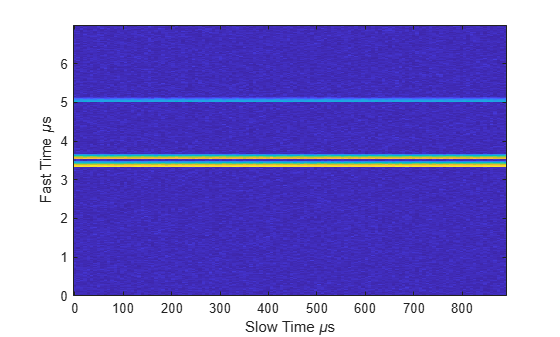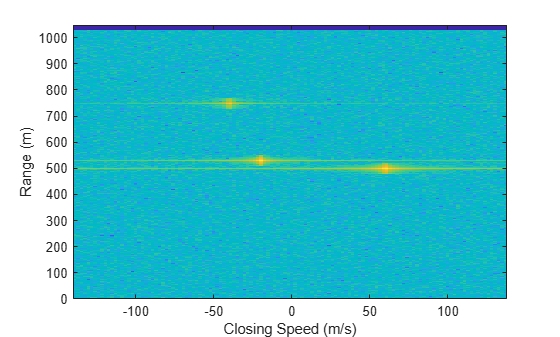# step

System object: phased.DopplerEstimator
Package: phased

Estimate target Doppler

## Syntax

dopest = step(estimator,resp,dopgrid,detidx)
[dopest,dopvar] = step(estimator,resp,dopgrid,detidx,noisepower)
[dopest,dopvar] = step(estimator,resp,dopgrid,detidx,clusterids)
[dopest,dopvar] = step(estimator,resp,dopgrid,detidx,noisepower,clusterids)

## Description

Note

Instead of using the step method to perform the operation defined by the System object™, you can call the object with arguments, as if it were a function. For example, y = step(obj,x) and y = obj(x) perform equivalent operations.

dopest = step(estimator,resp,dopgrid,detidx) estimates Doppler frequencies of detections derived from the range-Doppler response data, resp. Doppler estimates are computed for each detection position reported in detidx. The dopgrid argument sets the units for the Doppler dimension of the response data cube.

example

[dopest,dopvar] = step(estimator,resp,dopgrid,detidx,noisepower) also specifies the noise power. This syntax applies when you set the VarianceOutputPort property to true and the NoisePowerSource property to 'Input port'.
[dopest,dopvar] = step(estimator,resp,dopgrid,detidx,clusterids) also specifies the clusterids for the detections. This syntax applies when you set the ClusterInputPort property to true.
You can combine optional input and output arguments when their enabling properties are set. Optional inputs and outputs must be listed in the same order as the order of the enabling properties. For example, [dopest,dopvar] = step(estimator,resp,dopgrid,detidx,noisepower,clusterids).

Note

The object performs an initialization the first time the object is executed. This initialization locks nontunable properties and input specifications, such as dimensions, complexity, and data type of the input data. If you change a nontunable property or an input specification, the System object issues an error. To change nontunable properties or inputs, you must first call the release method to unlock the object.

## Input Arguments

expand all

Doppler estimator, specified as a phased.DopplerEstimator System object.

Example: phased.DopplerEstimator

Doppler-processed response data cube, specified as a complex-valued P-by-1 column vector, a complex-valued M-by-P matrix, or a complex-valued M-by-N-by-P array. M represents the number of fast-time or range samples. N is the number of spatial elements, such as sensor elements or beams. P is the number of Doppler bins.

The size of the first dimension of the input matrix can vary to simulate a changing signal length. A size change can occur, for example, in the case of a pulse waveform with variable pulse repetition frequency.

Data Types: single | double
Complex Number Support: Yes

Doppler grid values along the Doppler dimension of the resp argument, specified as a real-valued P-by-1 column vector. dopgrid defines the Doppler values corresponding to the Doppler dimension of the resp argument. Doppler values must be monotonically increasing and equally spaced. You can specify the grid values to be velocity or frequency. Units are in hertz or meters/sec.

Example: [-0.3,-0.2,-0.1,0,0.1,0.2,0.3]

Data Types: single | double

Detection indices, specified as a real-valued Nd-by-Q matrix. Q is the number of detections and Nd is the number of dimensions of the response data cube, resp. Each column of detidx contains the Nd indices of the detection in the response data cube.

To generate detection indices, you can use the phased.CFARDetector or phased.CFARDetector2D objects.

Data Types: single | double

Noise power at detection locations, specified as a positive scalar or real-valued 1-by-Q row vector of positive values. Q is the number of detections specified in detidx.

#### Dependencies

To enable this input argument, set the NoisePowerSource property to Input port.

Data Types: single | double

Cluster IDs, specified as a real-valued 1-by-Q row vector where Q is the number of detections specified in detidx. Each element of clusterids corresponds to a column in detidx. Detections with the same cluster ID belong to the same cluster.

#### Dependencies

To enable this input argument, set the ClusterInputPort property to true.

Data Types: single | double

## Output Arguments

expand all

Doppler estimates, returned as a real-valued K-by-1 column vector.

• When ClusterInputPort is false, Doppler estimates are computed for each detection location in the detidx argument. Then K equals the column dimension, Q, of detidx.

• When ClusterInputPort is true, Doppler estimates are computed for each cluster ID in the clusterids argument. Then K equals the number of unique cluster IDs, Q.

Data Types: single | double

Doppler estimation variance, returned as a positive, real-valued K-by-1 column vector, where K is the dimension of dopest. Each element of dopvar corresponds to an element of dopest. The estimator variance is computed using the Ziv-Zakai bound.

Data Types: single | double

## Examples

expand all

To estimate the range and speed of three targets, create a range-Doppler map using the phased.RangeDopplerResponse System object™. Then use the phased.RangeEstimator and phased.DopplerEstimator System objects to estimate range and speed. The transmitter and receiver are collocated isotropic antenna elements forming a monostatic radar system.

The transmitted signal is a linear FM waveform with a pulse repetition interval (PRI) of 7.0 μs and a duty cycle of 2%. The operating frequency is 77 GHz and the sample rate is 150 MHz.

fs = 150e6; c = physconst('LightSpeed'); fc = 77.0e9; pri = 7e-6; prf = 1/pri;

Set up the scenario parameters. The transmitter and receiver are stationary and located at the origin. The targets are 500, 530, and 750 meters from the radar along the x-axis. The targets move along the x-axis at speeds of –60, 20, and 40 m/s. All three targets have a nonfluctuating radar cross-section (RCS) of 10 dB. Create the target and radar platforms.

Numtgts = 3; tgtpos = zeros(Numtgts); tgtpos(1,:) = [500 530 750]; tgtvel = zeros(3,Numtgts); tgtvel(1,:) = [-60 20 40]; tgtrcs = db2pow(10)*[1 1 1]; tgtmotion = phased.Platform(tgtpos,tgtvel); target = phased.RadarTarget('PropagationSpeed',c,'OperatingFrequency',fc, ... 'MeanRCS',tgtrcs); radarpos = [0;0;0]; radarvel = [0;0;0]; radarmotion = phased.Platform(radarpos,radarvel);

Create the transmitter and receiver antennas.

txantenna = phased.IsotropicAntennaElement; rxantenna = clone(txantenna);

Set up the transmitter-end signal processing. Create an upsweep linear FM signal with a bandwidth of one half the sample rate. Find the length of the PRI in samples and then estimate the rms bandwidth and range resolution.

bw = fs/2; waveform = phased.LinearFMWaveform('SampleRate',fs, ... 'PRF',prf,'OutputFormat','Pulses','NumPulses',1,'SweepBandwidth',fs/2, ... 'DurationSpecification','Duty cycle','DutyCycle',0.02); sig = waveform(); Nr = length(sig); bwrms = bandwidth(waveform)/sqrt(12); rngrms = c/bwrms;

Set up the transmitter and radiator System object properties. The peak output power is 10 W and the transmitter gain is 36 dB.

peakpower = 10; txgain = 36.0; transmitter = phased.Transmitter( ... 'PeakPower',peakpower, ... 'Gain',txgain, ... 'InUseOutputPort',true); radiator = phased.Radiator( ... 'Sensor',txantenna,... 'PropagationSpeed',c,... 'OperatingFrequency',fc);

Set up the free-space channel in two-way propagation mode.

channel = phased.FreeSpace( ... 'SampleRate',fs, ... 'PropagationSpeed',c, ... 'OperatingFrequency',fc, ... 'TwoWayPropagation',true);

Set up the receiver-end processing. Set the receiver gain and noise figure.

collector = phased.Collector( ... 'Sensor',rxantenna, ... 'PropagationSpeed',c, ... 'OperatingFrequency',fc); rxgain = 42.0; noisefig = 1; receiver = phased.ReceiverPreamp( ... 'SampleRate',fs, ... 'Gain',rxgain, ... 'NoiseFigure',noisefig);

Loop over the pulses to create a data cube of 128 pulses. For each step of the loop, move the target and propagate the signal. Then put the received signal into the data cube. The data cube contains the received signal per pulse. Ordinarily, a data cube has three dimensions where the last dimension corresponds to antennas or beams. Because only one sensor is used, the cube has only two dimensions.

The processing steps are:

1. Move the radar and targets.

2. Transmit a waveform.

3. Propagate the waveform signal to the target.

4. Reflect the signal from the target.

5. Propagate the waveform back to the radar. Two-way propagation enables you to combine the return propagation with the outbound propagation.

7. Load the signal into the data cube.

Np = 128; dt = pri; cube = zeros(Nr,Np); for n = 1:Np [sensorpos,sensorvel] = radarmotion(dt); [tgtpos,tgtvel] = tgtmotion(dt); [tgtrng,tgtang] = rangeangle(tgtpos,sensorpos); sig = waveform(); [txsig,txstatus] = transmitter(sig); txsig = radiator(txsig,tgtang); txsig = channel(txsig,sensorpos,tgtpos,sensorvel,tgtvel); tgtsig = target(txsig); rxcol = collector(tgtsig,tgtang); rxsig = receiver(rxcol); cube(:,n) = rxsig; end

Display the data cube containing signals per pulse.

imagesc([0:(Np-1)]*pri*1e6,[0:(Nr-1)]/fs*1e6,abs(cube)) xlabel('Slow Time {\mu}s') ylabel('Fast Time {\mu}s') axis xyCreate and display the range-Doppler image for 128 Doppler bins. The image shows range vertically and speed horizontally. Use the linear FM waveform for match filtering. The image is here is the range-Doppler map.

ndop = 128; rangedopresp = phased.RangeDopplerResponse('SampleRate',fs, ... 'PropagationSpeed',c,'DopplerFFTLengthSource','Property', ... 'DopplerFFTLength',ndop,'DopplerOutput','Speed', ... 'OperatingFrequency',fc); matchingcoeff = getMatchedFilter(waveform); [rngdopresp,rnggrid,dopgrid] = rangedopresp(cube,matchingcoeff); imagesc(dopgrid,rnggrid,10*log10(abs(rngdopresp))) xlabel('Closing Speed (m/s)') ylabel('Range (m)') axis xyBecause the targets lie along the positive x-axis, positive velocity in the global coordinate system corresponds to negative closing speed. Negative velocity in the global coordinate system corresponds to positive closing speed.

Estimate the noise power after matched filtering. Create a constant noise background image for simulation purposes.

mfgain = matchingcoeff'*matchingcoeff; dopgain = Np; noisebw = fs; noisepower = noisepow(noisebw,receiver.NoiseFigure,receiver.ReferenceTemperature); noisepowerprc = mfgain*dopgain*noisepower; noise = noisepowerprc*ones(size(rngdopresp));

Create the range and Doppler estimator objects.

rangeestimator = phased.RangeEstimator('NumEstimatesSource','Auto', ... 'VarianceOutputPort',true,'NoisePowerSource','Input port', ... 'RMSResolution',rngrms); dopestimator = phased.DopplerEstimator('VarianceOutputPort',true, ... 'NoisePowerSource','Input port','NumPulses',Np);

Locate the target indices in the range-Doppler image. Instead of using a CFAR detector, for simplicity, use the known locations and speeds of the targets to obtain the corresponding index in the range-Doppler image.

detidx = NaN(2,Numtgts); tgtrng = rangeangle(tgtpos,radarpos); tgtspd = radialspeed(tgtpos,tgtvel,radarpos,radarvel); tgtdop = 2*speed2dop(tgtspd,c/fc); for m = 1:numel(tgtrng) [~,iMin] = min(abs(rnggrid-tgtrng(m))); detidx(1,m) = iMin; [~,iMin] = min(abs(dopgrid-tgtspd(m))); detidx(2,m) = iMin; end

Find the noise power at the detection locations.

ind = sub2ind(size(noise),detidx(1,:),detidx(2,:));

Estimate the range and range variance at the detection locations. The estimated ranges agree with the postulated ranges.

[rngest,rngvar] = rangeestimator(rngdopresp,rnggrid,detidx,noise(ind))
rngest = 3×1 499.7911 529.8380 750.0983 
rngvar = 3×1 10-4 × 0.0273 0.0276 0.2094 

Estimate the speed and speed variance at the detection locations. The estimated speeds agree with the predicted speeds.

[spdest,spdvar] = dopestimator(rngdopresp,dopgrid,detidx,noise(ind))
spdest = 3×1 60.5241 -19.6167 -39.5838 
spdvar = 3×1 10-5 × 0.0806 0.0816 0.6188 

## Version History

Introduced in R2017a